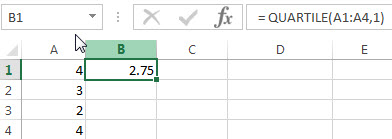# Excel QUARTILE Function

This post will guide you how to use the QUARTILE function with syntax and examples in Microsoft excel.

### Description

The Excel QUARTILE function returns the quartile from a supplied range of values. This function has been replaced with QUARTILE.EXC function and QUARTILE.INC function.

The QUARTILE function is a build-in function in Microsoft Excel and it is categorized as a Statistical Function.

The QUARTILE function is available in Excel 2016, Excel 2013, Excel 2010, Excel 2007, Excel 2003, Excel XP, Excel 2000, Excel 2011 for Mac.

### Syntax

The syntax of the QUARTILE function is as below:

`= QUARTILE (array,quart)`

Where the QUARTILE function arguments are:

• Array – This is a required argument.  The array or range of cells that contain numeric values for which you want to get the quartile value.
• Quart – This is a required argument. The quartile value that you want to return.  The value can be set in the following 5 values.
 If quart equals QUARTILE returns 0 Minimum value 1 First quartile (25th percentile) 2 Median value (50th percentile) 3 Third quartile (75th percentile) 4 Maximum value

Note:

• If the quart value is not an integer, and the QUARTILE function will truncate it.
• If the quart argument is empty, the QUARTILE function will return the #NUM! Error.
• If the quart value is small than 0 or greater than 4, the QUARTILE function will return the #NUM! Error.

### Excel QUARTILE Function Examples

The below examples will show you how to use Excel QUARTILE Function to get the quartile from a range of cells.

Example 1:  to get the first quartile of a given range of cells (A1:A4), using the following formula:

`= QUARTILE(A1:A4,1)`